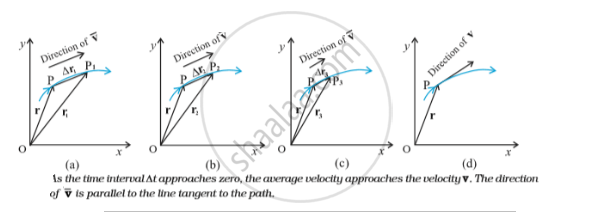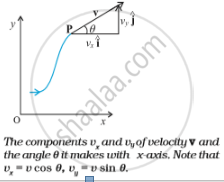# Cases of Uniform Velocity

## Notes

#### Velocity:

Velocity refers to a physical vector quantity which is described by both magnitude and direction. The magnitude or scalar absolute value of velocity is referred to as speed.
The average velocity (v bar) of an object is the ratio of the displacement and the corresponding time interval :
bar v = (Δr)/(Δt)=(Δx hat i + Δy hat j)/(Δt)=hat i (Δx)/(Δt) + hat j (y)/(Δt)
Or, bar v= bar v_x hat i + bar v_y bar j
v = lim_(Δt→0) (Δr)/(Δt)=(dr)/(dt)
The meaning of the limiting process can be easily understood with the help of the figures given below (a) to (d). In these figures, the thick line represents the path of an object, which is at P at time t. P_1, P_2 and P_3 represent the positions of the object after times Δt_1, Δt_2 and Δt_3. Δr_1, Δr_2 and Δr_3 are the displacements of the object in times Δt_1, Δt_2 and Δt_3 respectively. The direction of the average velocity bar v is shown in figures (a), (b) and (c) for three decreasing values of Δt, i.e., Δt_1, Δt_2 and Δt_3,(Δt_1>Δt_2 > Δt_3).As Δt →0, Δr →0 and  is along the tangent to the path [Fig.(d)]. Therefore, the direction of velocity at any point on the path of an object is tangential to the path at that point and is in the direction of motion.We can express v in a component form:
v=(dr)/(dt)=lim_(Δt→0)((Δx)/(Δt) hat i + (Δy)/(Δt) hat j)= hat i lim_(Δt→0)(Δx)/(Δt) + hat j lim_(Δt→0)(Δy)/(Δt)
Or, v =hat i (dx)/(dt) + hat j (dy)/(dt)= v_x hat i + v_y hat j.
where v_x=(dx)/(dt), v_y = (dy)/(dt)

So, if the expressions for the coordinates x and y are known as functions of time, we can use these equations to find vx(suffix x) and vy(suffix y). The magnitude of v is then v = sqrt(v_x^2+v_y^2)
and the direction of v is given by the angle θ, tanθ=(v_y)/(v_x), θ= tan^(-1)((v_y)/(v_x))

vx, vy and angle θ are shown in Fig. below for a velocity vector v at point p.If you would like to contribute notes or other learning material, please submit them using the button below.